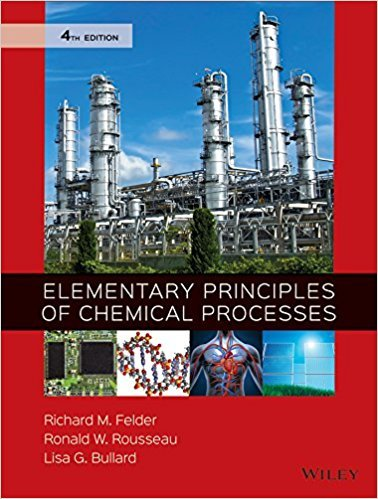×
×

# Solutions for Chapter 4.72: SUMMARY## Full solutions for Elementary Principles of Chemical Processes | 4th Edition

ISBN: 9780470616291Solutions for Chapter 4.72: SUMMARY

Solutions for Chapter 4.72
4 5 0 323 Reviews
21
5
##### ISBN: 9780470616291

This textbook survival guide was created for the textbook: Elementary Principles of Chemical Processes, edition: 4. Chapter 4.72: SUMMARY includes 1 full step-by-step solutions. Since 1 problems in chapter 4.72: SUMMARY have been answered, more than 45632 students have viewed full step-by-step solutions from this chapter. This expansive textbook survival guide covers the following chapters and their solutions. Elementary Principles of Chemical Processes was written by and is associated to the ISBN: 9780470616291.

Key Chemistry Terms and definitions covered in this textbook
• Acetal

A molecule containing two !OR or !OAr groups bonded to the same carbon

One-half the distance between the two nuclei in two adjacent atoms of the same element in a metal. For elements that exist as diatomic units, the atomic radius is one-half the distance between the nuclei of the two atoms in a particular molecule. (8.3)

• axis of symmetry

An axis about which a compound possesses rotational symmetry.

• changes of state

Transformations of matter from one state to a different one, for example, from a gas to a liquid. (Section 1.3)

• chemical equilibrium.

A state in which the rates of the forward and reverse reactions are equal. (14.1)

• diastereomers

Stereoisomers that are not mirror images of one another.

• Double helix

A type of secondary structure of DNA molecules in which two anti parallel polynucleotide strands are coiled in a right-handed manner about the same axis

• f-block metals

Lanthanide and actinide elements in which the 4f or 5f orbitals are partially occupied. (Section 6.9)

• Formal charge

The charge on an atom in a polyatomic ion or molecule

• frequency factor (A)

A term in the Arrhenius equation that is related to the frequency of collision and the probability that the collisions are favorably oriented for reaction. (Section 14.5)

• joule (J)

The SI unit of energy, 1 kg@m2 >s2. A related unit is the calorie: 4.184 J = 1 cal. (Section 5.1)

• mass-to-charge ratio(m/z)

Thedetermining factor by which ions are separatedfrom each other in mass spectrometry.

• mixture

A combination of two or more substances in which each substance retains its own chemical identity. (Section 1.2)

• monomers

Molecules with low molecular weights, which can be joined together (polymerized) to form a polymer. (Section 12.8)

• oils

Triglycerides that are liquids at room temperature.

• probability density 1c22

A value that represents the probability that an electron will be found at a given point in space. Also called electron density. (Section 6.5)

• regiochemistry

A term describing a consideration that must be taken into account for a reaction in which two or more constitutional isomers can be formed.

• solubility-product constant (solubility product)1Ksp2

An equilibrium constant related to the equilibrium between a solid salt and its ions in solution. It provides a quantitative measure of the solubility of a slightly soluble salt. (Section 17.4)

• Telechelic polymer

A polymer in which its growing chains are terminated by formation of new functional groups at both ends of its chains. These new functional groups are introduced by adding reagents, such as CO2 or ethylene oxide, to the growing chains.

• Woodward-Fieser rules

Rules for predicting the wavelength of maximum absorption for a compound with extended conjugation.

×